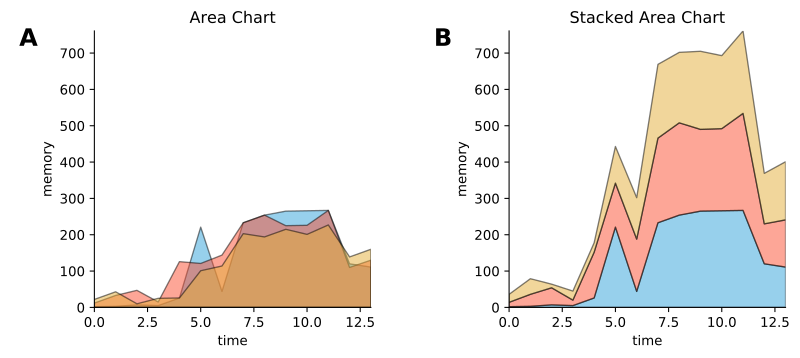Area chart¶

Most examples work across multiple plotting backends equivalent, this example is also available for:

In :
import numpy as np
import holoviews as hv
from holoviews import opts

hv.extension('matplotlib')
hv.output(fig='svg')Declaring data¶

In :
# create some example data
python=np.array([2, 3, 7, 5, 26, 221, 44, 233, 254, 265, 266, 267, 120, 111])
pypy=np.array([12, 33, 47, 15, 126, 121, 144, 233, 254, 225, 226, 267, 110, 130])
jython=np.array([22, 43, 10, 25, 26, 101, 114, 203, 194, 215, 201, 227, 139, 160])

dims = dict(kdims='time', vdims='memory')
python = hv.Area(python, label='python', **dims)
pypy   = hv.Area(pypy,   label='pypy',   **dims)
jython = hv.Area(jython, label='jython', **dims)

Plot¶

In :
overlay = (python * pypy * jython).opts(opts.Area(alpha=0.5))
overlay.relabel("Area Chart") + hv.Area.stack(overlay).relabel("Stacked Area Chart")
Out: